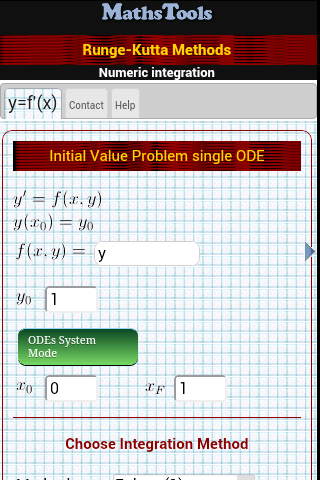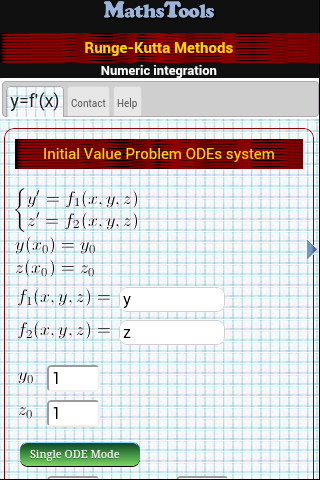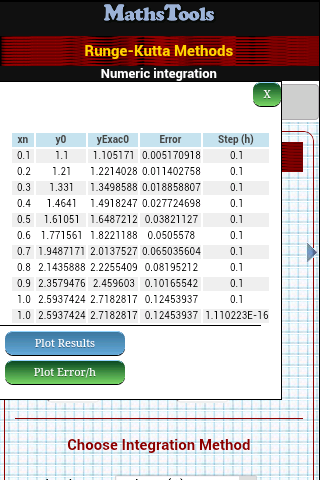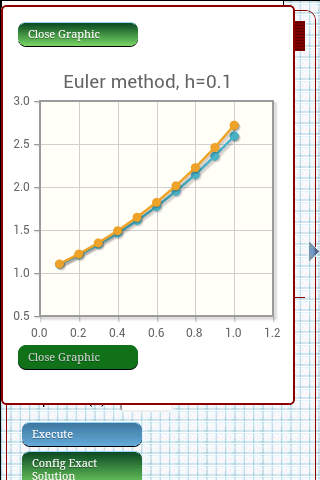Runge-Kutta methods: The Android App for Runge-Kutta methods

Basic concepts

Runge-Kutta Methods is an application to solve numerically Ordinary Differential Equations and system of Ordinary Differential Equations in Android devices.

Runge-Kutta Methods Supports a large number of methods with diferent integration order from the order 1 in the Euler method to complex Fehlberg and Dormand Prince several methods

Problems in the form

y' = f(x, y)
y(x0)=y 0

Is possible to solve numerically withRunge-Kutta MethodsScreen for input a single ODE Screen to input an ODEs systemData output Screen Data plotting Screen

Was useful? want add anything?

Post here

Post from other users

Post here